# Mixed fractions calculator

The calculator performs basic and advanced operations with mixed numbers, fractions, integers, decimals. Mixed fractions are also called mixed numbers. A mixed fraction is a whole number and a proper fraction combined, i.e. one and three-quarters. The calculator evaluates the expression or solves the equation with step-by-step calculation progress information. Solve problems with two or more mixed numbers fractions in one expression.

## Result:

### 22/3 - 11/4 = 17/12 = 1 5/12 ≅ 1.4166667

Spelled result in words is one and five twelfths (or seventeen twelfths).

### Calculation steps

1. Conversion a mixed number 2 2/3 to a improper fraction: 2 2/3 = 2 2/3 = 2 · 3 + 2/3 = 6 + 2/3 = 8/3

To find new numerator:
a) Multiply the whole number 2 by the denominator 3. Whole number 2 equally 2 * 3/3 = 6/3
b) Add the answer from previous step 6 to the numerator 2. New numerator is 6 + 2 = 8
c) Write a previous answer (new numerator 8) over the denominator 3.

Two and two thirds is eight thirds
2. Conversion a mixed number 1 1/4 to a improper fraction: 1 1/4 = 1 1/4 = 1 · 4 + 1/4 = 4 + 1/4 = 5/4

To find new numerator:
a) Multiply the whole number 1 by the denominator 4. Whole number 1 equally 1 * 4/4 = 4/4
b) Add the answer from previous step 4 to the numerator 1. New numerator is 4 + 1 = 5
c) Write a previous answer (new numerator 5) over the denominator 4.

One and one quarter is five quarters
3. Subtract: 8/3 - 5/4 = 8 · 4/3 · 4 - 5 · 3/4 · 3 = 32/12 - 15/12 = 32 - 15/12 = 17/12
For adding, subtracting, and comparing fractions, it is suitable to adjust both fractions to a common (equal, identical) denominator. The common denominator you can calculate as the least common multiple of both denominators - LCM(3, 4) = 12. In practice, it is enough to find the common denominator (not necessarily the lowest) by multiplying the denominators: 3 × 4 = 12. In the next intermediate step, the fraction result cannot be further simplified by canceling.
In words - eight thirds minus five quarters = seventeen twelfths.

#### Examples:

sum of two mixed numbers: 1 3/4 + 2 3/8
addition of three mixed numbers: 1 3/8 + 6 11/13 + 5 7/8
addition of two mixed numbers: 2 1/2 + 4 2/3
subtracting two mixed numbers: 7 1/2 - 5 3/4
multiplication of mixed numbers: 3 3/4 * 2 2/5
comparing mixed numbers: 3 1/4 2 1/3
changing improper fraction to mixed number: 9/4
What is 3/4 as a mixed number: 3/4
subtracting mixed number and fraction: 1 3/5 - 5/6
sum mixed number and an improper fraction: 1 3/5 + 11/5

## Mixed fractions in word problems:

• GavinGavin had 3 gallons of orange juice ready to serve at the breakfast party. His family drank 2 ⅛ gallons. How much is left? Show your work with a numberline, area model or grid.
• Difference mixed fractionsWhat is the difference between 4 2/3 and 3 1/6?
• Cups of punchCyka made 6 19/20 cups of punch punch at two different types of juice in it. If the punch had 4 1/5 cups of one type of juice how many cups of the other type of juice did it have?
• Sum of 18Sum of two fractions is 4 3/7. If one of the fractions is 2 1/5 find the other one .
• Sum of mixedThe sum of two mixed numbers is 15½. One of the number is 5 ¾, what is the other?
• Equation with mixed 2A number, X, is subtracted from 8 1/4. The result is 12 3/5. What is the value of X?
• Light-years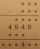Planet X is 10 light-years away from Earth. Planet Y is 8 1/2 light-years away from Earth. How much farther away is Planet X?
• Sum of 20Sum of the two numbers is 15 1/6. If one of the numbers is 2 2/3, find the other number.
• Denissa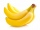Denissa has 18 9/10 kilos of bananas to sell if she was able to sell 15 ⅗. How many kilos of bananas were left?
• TyronTyron attended his online class 2 hours a day. He already attend 1 3/4 hours, how many more hours will Tyron attend his class?
• Bike pathBill was riding his bike on a bike path that was 5 1/4 miles long. He rode his bike awhile and stopped for s drink or water. Then he rode 2 7/10 miles and was at the end of the path. How far had Bill ridden before he stopped for water?
• Mixed numbersRewrite mixed numbers, so the fractions have the same denominator: 5 1/5 - 2 2/3
• Hannah and Emily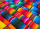Hannah and Emily need to use  4 1/4  feet of ribbon to finish an art project. Hannah has 2 3/4 feet of ribbon and Emily has 1 3/4 feet of ribbon. How much ribbon will they have left over after they finish their art project?
• The chef 2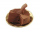The chef in the restaurant used 19/20  cup of chocolate chips and 1 1/10 cups of cocoa powder for the cake. How much more cocoa powder did the chef use than the chocolate chips?
• RegroupingSubtract mixed number with regrouping: 11 17/20- 6 19/20What is 4 1/2+2/7-213/14?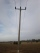Marcos wants to buy grass seed to cover his whole lawn, except for the pool. The pool is 7 1/2 m by 3 1/4 m. Find the area the grass seed needs to cover. Dimensions of the lawn are 50 m x 150 m.